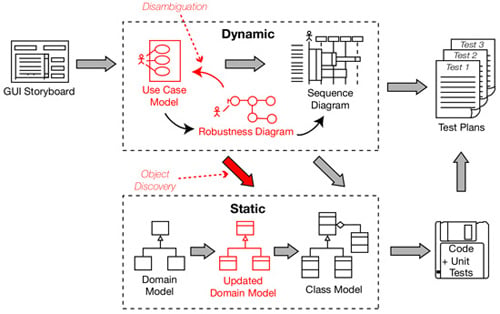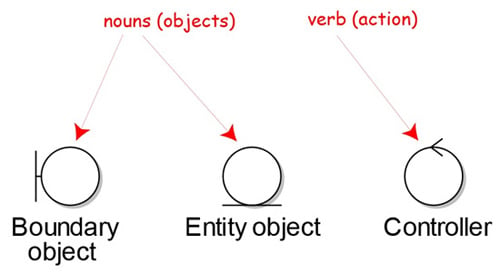# Close the gap between analysis and design

## Get robust

Book extract, part 3 To get from use cases to detailed design (and then to code), you need to link your use cases to objects. The technique we describe in this chapter, robustness analysis, helps you to bridge the gap from analysis to design by doing exactly that.

As in our first two extracts (here and here), let's start by mapping where we are in the ICONIX process. This time, how robustness analysis fits into the process.Robustness analysis - brings it all together

Robustness analysis in nutshell is a way of analyzing your use-case text and identifying a first-guess set of objects for each use case. These are classified into boundary objects, entity objects, and controllers (which are often more like functions than objects) - see the next diagram for more.

A robustness diagram is an object picture of a use case. The robustness diagram and the use-case text have to match precisely, so the robustness diagram forces you to tie the use-case text to the objects. This enables you to drive object-oriented designs forward from use cases, and this is really the "magic" of robustness analysis.Robustness diagram symbols